General, Organic, and Biological Chemistry: General, Organic, and Biological Chemistry Final Flashcards

Set Details Share
created 8 years ago by imaninja05
653 views
updated 8 years ago by imaninja05
Subjects:
chemistry
Page to share:
Embed this setcancel
COPY
code changes based on your size selection
Size:
X
1

The amount of space occupied by a substance is its

Volume

2

The measurement of the gravitational pull on an object is its

Weight

3

The energy stored in the chemical bonds of a carbohydrate molecule is

Potential energy

4

The energy of motion is referred to as

Kinetic energy

5

Physical Changes consist of

• Freezing water to make ice cubes
• tearing a piece of aluminum foil
• boiling water for soup
• melting gold to make jewelry
6

Specific Heat

Amount of energy needed to raise the temperature of 1 g of the substance by 1 degree Celsius.

7

Air is a(n)

Homogenous mixture

8

When a solid is converted directly to a gas, the change of state is called

Sublimation

9

What is an example of a pure substance?

Ethyl alcohol

10

What is the term that describes a liquid changing to a vapor at a temperature less than its boiling point?

Evaporation

11

Formula for density is

D = Mass/Volume

12

Formula for converting degrees F to degrees C

C = (F - 32)/ 1.8

13

Formula for converting degrees C to degrees F

F = 1.8(C) + 32

14

Formula for converting degrees C to degrees K

K = C + 273.15

15

1 inch is = to

2.54 cm

16

1 Liter is = to

1.06 qt

17

1 kg is = to

2.20 lbs

18

What is specific gravity?

SG = Density/ Density of H2O (1.00g/mL)

19

Characteristics of Alkali Metals are

Malleable

Ductile

Good conductors of heat and electricity

20

The smallest particle of an element that retains the characteristics of the element is a(n)

Atom

21

Subatomic particle

A proton has a positive charge and a mass of approximately 1 amu (atomic mass unit)

22

What is the mass number?

The mass number corresponds to the mass of the atom, that is obtained by adding the number of protons and neutrons present. The mass numbers appears under the element symbol in the elements table.

23

The atomic size of an atom

Increases going down within a group

24

Ionization energy is

The energy needed to remove the least tightly bound electron.

25

The octet rule indicates that

Atoms lose, gain, or share valence electrons to have 8 valence electrons.

26

Every atom/ion of a particular element has the same number of

Protons

27

Double and triple bonds form because

Single covalent bonds do not give all of the atom in the molecule 8 valence electrons.

28

Polar Covalent Bond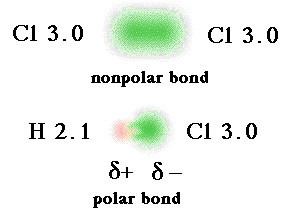The greater the electronegativity difference, the more ionic the bond is.

29

Nonpolar Covalent BondsEqual sharing of the bond electrons, arise when the electronegativities of the two atoms are equal

30

In ionic compounds,_____lose their valence electrons to form positively charged_____.

metals; cations

31

The main interaction between molecules of hydrogen are examples of

Dispersion forces

32

Dispersion Forces

Is a temporary attractive force that results when the electrons in two adjacent atoms occupy positions that make the atoms form temporary dipoles

33

Heat of Fusion

The energy that must be removed to turn 1 g of water at 0 degrees Celsius to 1 g of ice at 0 degrees Celsius.

34

Heat of Vaporization

The energy that must be added to turn 1 g of water at 100 degrees Celsius to 1 g of steam at 100 degrees Celsius.

35

How to calculate atomic mass average

1. Multiply given mass amounts by percentage sample. (mass 13.00 amu) makes up 1.07%. (mass 12.00 amu) makes up 98.93% 13.00(1.07%) = 13.91 12.00(98.93%) = 1187.16
2. Add the totals and divide by 100.
3. Sig Fig is 4 so answer is 12.01 from 1201.07
36

Formula to calculate calories needed to raise the temperature

Energy = SH x mass x temperature change

example: 1.00 cal/g degrees Celsius(100.00 g)(100-25) = 7500 calories about 7.50x10 to the 3rd power

37

Electron Affinity

The energy needed to remove the least tightly bound electron from an atom.

38

Trend of Electron Affinity

Increases across a period and decreases down a group.

39

A chemical equation is balanced when

The number of each element on the reactant side equals the number of each element on the product side.

40

Combination reactions

Two or more elements or compounds bond to form one product.

41

Decomposition reactions

A reactant splits into two or more simpler products.

42

Single replacement reactions

A reacting element switches place with an element in the other reacting compound.

43

Double replacement reactions

The positive ions in the reacting compounds switch places.

44

Combustion reactions

A carbon-containing compound that is the fuel burns in oxygen from the air to produce carbon dioxide, water, and energy in the form of heat or flame.

45

Oxdation

Loss of electrons.

46

Reduction

Gain of electrons.

47

Mole (Avogardo's Number)

One mole of any element always contains 6.02 x 1023 atoms.

Example: Convert 4.00 moles of Fe atoms.

4.00 moles Fe atoms x 6.02 x 1023 Fe atoms /1 mole Fe atoms = 2.41 x1024 Fe atoms.

48

Endothermic Reaction

Energy is absorbed by the system.

49

Kinetic Theory of Gas

1. Composed of very small particles
2. Gas particles move rapidly
3. Gas particles do not attract or repel one another
4. Gas particles move faster when the temperature increases
50

Pressure

The forces of gas particles against the walls of a container.

51

Relationship between atm(atmospheres) and mmHg(millimeter of mercury)

1 atm = 760 mmHg

52

Charles's Law

The volume of gas decreases when the temperature decreases.

Formula: V1/T1 =V2/T2

53

Boyle's Law

The pressure and volume of a gas have an inverse relationship, when temperature is held constant.

Formula: P1V1 = P2V2

54

Gay-Lussac's Law

The relationship between the pressure and temperature of a fixed mass of gas kept at a constant volume.

Formula: P1/T1 = P2/T2

55

The volume of a gas is directly related to the number of moles of a gas when pressure and temperature are not changed.

Formula: V1/n1 = V2/n2

56

Molar volume of a gas at STP?

22.4 L/mol

57

Combined Gas Law

All the pressure-volume-temperature relationships for gasses combined to make a single relationship.

Formula: P1V1/T1 = P2V2/T2

58

Ideal Gas Law

The combination of the four properties used in the measurement of a gas-pressure (P), volume (V), temperature (T), and amount (n) to give a single expression.

Formula: PV = nRT

Rearranged, shows the 4 gas properties equal a constant R.

Formula: PV/nT = R

59

Relationship between atm(atmospheres) and torr( SI unit of pressure)

1 atm = 760 torr

60

Relationship between moles of gas and Liters

1 mole of gas (STP) = 22.4 L

61

Relationship between Constant R and the 4 gas laws.

R = (1.00 atm)(22.4)/(1.00 mole)(273 K) = 0.0821 L x atm/ mole x K

62

In a solution, the solvent

Can be solid, liquid, or gas.

63

Oil does not dissolve in water because

oil is nonpolar

64

When NaCl dissolves in water

The Na+ ions are attracted to the partially negative oxygen atoms of the water molecule.

65

In water, a substance that ionizes completely in a solution is called a

Strong electrolyte

66

To calculate equivalents present of moles in a charged element you would

Multiply the amount of moles by the elements charge

Example: 5.0 moles of Ca2+ is 5.0 x 2 = 10 Eq

67

Calculate the concentration of a solution in mass/volume %

68

Hypertonic

More solute than the other.

69

Solvent

Doing the dissolving.

70

Solute

Being dissolved.

71

Weak Electrolyte

A compound that is dissolved in water mostly a molecules.

72

Solubility

The amount of a solute that can be dissolved in a given amount of solvent.

73

Unsaturated solution

Rapidly dissolving when added to a solvent.

74

According to the Bronsted-Lowry definition,

A base is a proton acceptor

75

Saturated solution

A solution that contains all the solute that can dissolve which is the rate at which solute dissolves become equal to the rate at which solids form, also known as recrystallization.

76

Buffer

A weak acid/base and its conjugate base/acid salt which reacts with small amounts of an acid or base to maintain pH at a constant value.

77

Signs that a chemical change is taking place

1. Solid dissolves
2. Gas formed
3. Precipitate formed
4. Heat released or absorbed
5. Color change

Examples: Changes in size or shape, changes in state, solid -> liquid -> gas

78

Osmotic Pressure

The pressure which is caused by 2 solutions with different solute concentrations separated by a semipermeable membrane.

It also causes water to flow from more concentrated to less concentrated.

79

Hypotonic

Less solute than the other.

80

Activation energy

The minimum amount of energy required to break the bonds between atoms of reactants.

81

Rate of reaction

Is determined by measuring the amount of a reactant used up, or the amount of a product formed, in a certain period of time.

82

Factors that affect the rate of reaction

1. Temperature-higher temps. increase in kinetic energy makes the reacting molecules move faster.
2. Concentration of reactants-increases when the concentration of reactants increase.
3. Catalysts-another way to speed up a reaction is to lower the activation energy
83

Chemical Equilibrium

The rate of the forward reaction is equal to the rate of the reverse reaction.

No further changes occur in the concentrations of the reactants or products; the forward and reverse reactions continue at equal rates.

84

Equilibrium Constant Expression

Multiplies the concentrations of the products ad divides by the concentrations of the reactants.

85

Homogenous equilibrium

A reaction in which all the reactants and products are gases.

86

Heterogeneous equilibrium

When the reactants and products are in 2 or more physical states.

87

A reaction with a large K c

Contains mostly products.

Examples: 2 x 1011, 1.1 x 107, 1.6 x 102

88

A reaction with a small K c

Contains mostly reactants.

Examples: 1.2 x 10-2, 2 x 10-9, 4.7 x 10-14

89

Le Chatelier's principle

States than when a system at equilibrium is disturbed, the system will shift in the direction that will reduce that stress.

90

Acids

• Substances that produces hydrogen ions (H+) when they dissolve in water.
• When dissolved in water it produces a hydrogen ion and a simple nonmetal anion.
• Prefix: hydro
• Ending in ide is changed to ic acid
• Polyatomic anion that ends in ite is replaced with ous acid
• Taste sour
• pH less than 7
• Turns blue litmus to red
• Acids neutralize bases producing salt and water
• Proton donors ( Proton H+ ion = hydrogen ion)
• Increases H ion concentration in solution
• Are electrolytes
91

Bases

• Are ionic compounds that dissociate into cations and hydroxide ions (OH-) when dissolved in water
• Taste bitter, chalky
• pH greater than 7
• Turn red litmus blue
• Neutralize acids producing a salt and water
• Proton acceptors (H+ = hydrogen ion)
• Increase OH- ion concentration in solution
• Are electrolytes
• Feel slippery
92

Conjugate acid-Base Pairs

Consist of molecules or ions related by the loss of one H+ by an acid and the gain of one H+ by a base.

93

Identify the conjugate acid-base pairs:

HBr(aq) + NH3(aq) ----> Br-(aq) + NH4 +(aq)

• Acting as a Bronsted-Lowry acid, HBr loses 1 H+ to form Br-, which is a conjugate base.
• The NH3, acting as a Bronsted-Lowry base, gains 1 H+ to form its conjugate acid NH4 +.
• The conjugate acid-base pairs are HBr/Br- and NH4 +/NH3
94

Dissociation

An acid or base separates into ions in water.

95

Strong acids

Donate hydrogen ions so easily that their dissociation in water is nearly complete.

96

Weak acids

Dissociates slightly in water, which means only a small percentage of H+ is transferred from a weak acid to H2O, forming only a small amount of H3O+

97

What is the pH of a neutral solution?

7

98

To find the pH of any solution, you would

Identify the solution containing an acid or base.

Significant Figures.

Examples:

.010 M HCl (acid H3O+) [H3O+] = 1.0 x 10-2

0.0100 M NaOH (base OH-) [OH-] = 1.0 x 10-2 pH = 14 14-2 = 12.00

99

Alpha emitter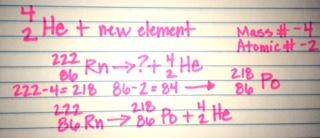100

Beta emitter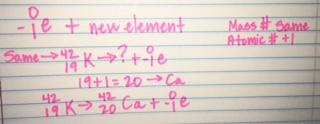101

Positron emitter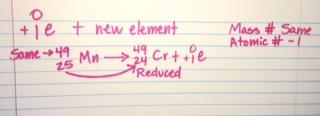102

Gamma emitter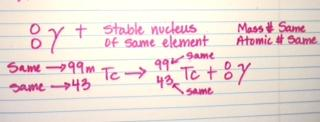103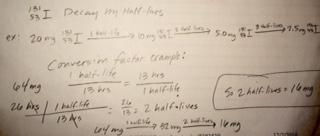104

• Protective clothing
• Increase distance from source
105

Hydroxide

OH-

106

Ammonium

NH4 +

107

Nitrate

NO3 -

108

Nitrite

NO2 -

109

Perchlorate

ClO4 -

110

Chlorate

ClO3 -

111

Chlorite

ClO2 -

112

Hypochlorite

ClO-

113

Carbonate

CO3 -2

114

Hydrogen Carbonate (bicarbonate)

HCO3 -

115

Cyanide

CN-

116

Acetate

C2H3O2 -

117

Sulfate

SO4 -2

118

Hydrogen Sulfate (bisulfate)

HSO4 -

119

Phosphate

PO 4 -3

120

Hydrogen Phosphate

HPO4 -2

121

Dihydrogen Phosphate

H2 PO4 -2

122

Phosphate

PO3 -3